Home
Hostname: page-component-55597f9d44-ssw5r Total loading time: 0.214 Render date: 2022-08-14T11:03:57.939Z Has data issue: true Feature Flags: { "shouldUseShareProductTool": true, "shouldUseHypothesis": true, "isUnsiloEnabled": true, "useRatesEcommerce": false, "useNewApi": true } hasContentIssue true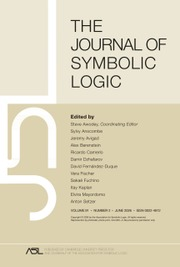The Journal of Symbolic Logic

# ON OBDD-BASED ALGORITHMS AND PROOF SYSTEMS THAT DYNAMICALLY CHANGE THE ORDER OF VARIABLES

Published online by Cambridge University Press:  22 June 2020

## Abstract

In 2004 Atserias, Kolaitis, and Vardi proposed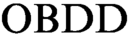$\text {OBDD}$ -based propositional proof systems that prove unsatisfiability of a CNF formula by deduction of an identically false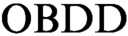$\text {OBDD}$ from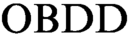$\text {OBDD}$ s representing clauses of the initial formula. All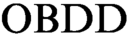$\text {OBDD}$ s in such proofs have the same order of variables. We initiate the study of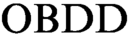$\text {OBDD}$ based proof systems that additionally contain a rule that allows changing the order in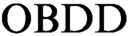$\text {OBDD}$ s. At first we consider a proof system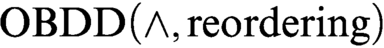$\text {OBDD}(\land , \text{reordering})$ that uses the conjunction (join) rule and the rule that allows changing the order. We exponentially separate this proof system from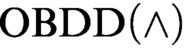$\text {OBDD}(\land )$ proof system that uses only the conjunction rule. We prove exponential lower bounds on the size of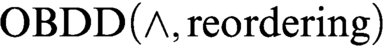$\text {OBDD}(\land , \text{reordering})$ refutations of Tseitin formulas and the pigeonhole principle. The first lower bound was previously unknown even for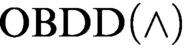$\text {OBDD}(\land )$ proofs and the second one extends the result of Tveretina et al. from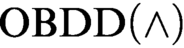$\text {OBDD}(\land )$ to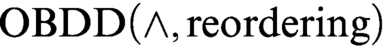$\text {OBDD}(\land , \text{reordering})$ .

In 2001 Aguirre and Vardi proposed an approach to the propositional satisfiability problem based on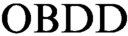$\text {OBDD}$ s and symbolic quantifier elimination (we denote algorithms based on this approach as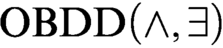$\text {OBDD}(\land , \exists )$ algorithms). We augment these algorithms with the operation of reordering of variables and call the new scheme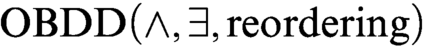$\text {OBDD}(\land , \exists , \text{reordering})$ algorithms. We notice that there exists an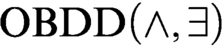$\text {OBDD}(\land , \exists )$ algorithm that solves satisfiable and unsatisfiable Tseitin formulas in polynomial time (a standard example of a hard system of linear equations over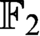$\mathbb {F}_2$ ), but we show that there are formulas representing systems of linear equations over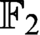$\mathbb {F}_2$ that are hard for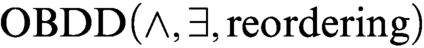$\text {OBDD}(\land , \exists , \text{reordering})$ algorithms. Our hard instances are satisfiable formulas representing systems of linear equations over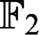$\mathbb {F}_2$ that correspond to checksum matrices of error correcting codes.

## MSC classification

Type
Articles
Information
The Journal of Symbolic Logic , June 2020 , pp. 632 - 670
© The Association for Symbolic Logic 2020

## Access options

Get access to the full version of this content by using one of the access options below. (Log in options will check for institutional or personal access. Content may require purchase if you do not have access.)

## Footnotes

The present paper is an expansion of an earlier conference paper . It includes the contents of  augmented with all the proofs that were omitted in the conference version. In addition, the paper contains a new deterministic construction of a hard example for$\text {OBDD}(\land , \exists )\$ algorithms.

## References

Aguirre, A. S. M. and Vardi, M. Y., Random 3-sat and bdds: The plot thickens further, Principles and Practice of Constraint Programming—CP 2001 (Walsh, T., editor), Lecture Notes in Computer Science, vol. 2239, Springer, Berlin, 2001, pp. 121136.CrossRefGoogle Scholar
Alon, N. and Chung, F. R. K., Explicit construction of linear sized tolerant networks . Discrete Mathematics, vol. 306 (2006), no. 10–11, pp. 10681071.CrossRefGoogle Scholar
Atserias, A., Kolaitis, P. G., and Vardi, M. Y., Constraint propagation as a proof system, Principles and Practice of Constraint Programming—CP 2004 (Wallace, M., editor), Lecture Notes in Computer Science, vol. 3258, Springer, Berlin, 2004, pp. 7791.CrossRefGoogle Scholar
Bonet, M. L., Pitassi, T., and Raz, R., Lower bounds for cutting planes proofs with small coefficients , this Journal, vol. 62 (1997), no. 3, pp. 708728.Google Scholar
Buss, S., Itsykson, D., Knop, A., and Sokolov, D., Reordering rule makes OBDD proof systems stronger, 33rd Computational Complexity Conference, CCC 2018 (Servedio, R. A., editor), Leibniz International Proceedings in Informatics, Schloss Dagstuhl, Dagstuhl, 2018, pp. 16:116:24.Google Scholar
Cheeger, J., A lower bound for the smallest eigenvalue of the Laplacian . Problems in Analysis, vol. 78 (1970), p. 195.Google Scholar
Chén, W. and Zhang, W., A direct construction of polynomial-size OBDD proof of pigeon hole problem . Information Processing Letters, vol. 109 (2009), no. 10, pp. 472477.CrossRefGoogle Scholar
Cook, S. A. and Reckhow, R. A., The relative efficiency of propositional proof systems , this Journal, vol. 44 (1979), no. 1, pp. 3650.Google Scholar
Cook, W. J., Coullard, C. R., and Turán, G., On the complexity of cutting-plane proofs . Discrete Applied Mathematics, vol. 18 (1987), no. 1, pp. 2538.CrossRefGoogle Scholar
Duris, P., Hromkovic, J., Jukna, S., Sauerhoff, M., and Schnitger, G., On multi-partition communication complexity . Information and Computation, vol. 194 (2004), no. 1, pp. 4975.CrossRefGoogle Scholar
Friedman, L. and Xu, Y., Exponential lower bounds for refuting random formulas using ordered binary decision diagrams, Computer Science—Theory and Applications (Bulatov, A. A. and Shur, A. M., editors), Lecture Notes in Computer Science, vol. 7913, Springer, Berlin, 2013, pp. 127138.CrossRefGoogle Scholar
Gallager, R. G., Low-density parity-check codes . Institute of Electrical and Electronics Engineers. Transactions on Information Theory, vol. 8 (1962), no. 1, pp. 2128.CrossRefGoogle Scholar
Groote, J. F. and Zantema, H., Resolution and binary decision diagrams cannot simulate each other polynomially . Discrete Applied Mathematics, vol. 130 (2003), no. 2, pp. 157171.CrossRefGoogle Scholar
Guruswami, V., List decoding from erasures: bounds and code constructions . Institute of Electrical and Electronics Engineers. Transactions on Information Theory, vol. 49 (2003), no. 11, pp. 28262833.CrossRefGoogle Scholar
Hoory, S., Linial, N., and Wigderson, A., Expander graphs and their applications . Bulletin of the American Mathematical Society, vol. 43 (2006), no. 4, pp. 439561.CrossRefGoogle Scholar
Impagliazzo, R., Pitassi, T., and Urquhart, A., Upper and lower bounds for tree-like cutting planes proofs, Proceedings of the Ninth Annual IEEE Symposium on Logic in Computer Science , Institute of Electrical and Electronics Engineers, Piscataway, NJ, 1994, pp. 220228.CrossRefGoogle Scholar
Itsykson, D., Knop, A., Romashchenko, A. E., and Sokolov, D., On OBDD-based algorithms and proof systems that dynamically change order of variables, 34th Symposium on Theoretical Aspects of Computer Science (Vollmer, H. and Vallée, B., editors), Leibniz International Proceedings in Informatics, Schloss Dagstuhl, Dagstuhl, 2017, pp. 43:143:14.Google Scholar
Krajícek, J., Interpolation theorems, lower bounds for proof systems, and independence results for bounded arithmetic , this Journal, vol. 62 (1997), no. 2, pp. 457486.Google Scholar
Krajícek, J., An exponential lower bound for a constraint propagation proof system based on ordered binary decision diagrams , this Journal, vol. 73 (2008), no. 1, pp. 227237.Google Scholar
Kushilevitz, E. and Nisan, N., Communication Complexity, Cambridge University Press, Cambridge, 1997.Google Scholar
Lubotzky, A., Phillips, R., and Sarnak, P., Ramanujan graphs . Combinatorica, vol. 8 (1988), no. 3, pp. 261277.CrossRefGoogle Scholar
Meinel, C. and Slobodova, A., On the complexity of constructing optimal ordered binary decision diagrams . Proceedings of Mathematical Foundations of Computer Science, vol. 841 (1994), pp. 515524.Google Scholar
Meinel, C. and Theobald, T., Algorithms and Data Structures in VLSI Design: OBDD—Foundations and Applications, Springer, New York, 1998.CrossRefGoogle Scholar
Pan, G. and Vardi, M. Y., Symbolic techniques in satisfiability solving . Journal of Automated Reasoning, vol. 35 (2005), no. 1–3, pp. 2550.CrossRefGoogle Scholar
Segerlind, N., Nearly-exponential size lower bounds for symbolic quantifier elimination algorithms and OBDD-based proofs of unsatisfiability, preprint, 2007, arXiv:cs/0701054.Google Scholar
Segerlind, N., On the relative efficiency of resolution-like proofs and ordered binary decision diagram proofs . Electronic Colloquium on Computation Complexity, vol. 126 (2007), p. 23.Google Scholar
Tveretina, O., Sinz, C., and Zantema, H., An exponential lower bound on OBDD refutations for pigeonhole formulas. Proceedings Fourth Athens Colloquium on Algorithms and Complexity (E. Markakis and I. Milis, editors), Electronic Proceedings in Theoretical Computer Science, vol. 4, pp. 13–21.CrossRefGoogle Scholar
Urquhart, A., Hard examples for resolution . Journal of the ACM, vol. 34 (1987), no. 1, pp. 209219.CrossRefGoogle Scholar
Wegener, I., Branching Programs and Binary Decision Diagrams, SIAM, New York, 2000.CrossRefGoogle Scholar
Zémor, G., On expander codes . IEEE Transactions on Information Theory, vol. 47 (2001), no. 2, pp. 835837.CrossRefGoogle Scholar
1
Cited by

# Save article to Kindle

Note you can select to save to either the @free.kindle.com or @kindle.com variations. ‘@free.kindle.com’ emails are free but can only be saved to your device when it is connected to wi-fi. ‘@kindle.com’ emails can be delivered even when you are not connected to wi-fi, but note that service fees apply.

Find out more about the Kindle Personal Document Service.

ON OBDD-BASED ALGORITHMS AND PROOF SYSTEMS THAT DYNAMICALLY CHANGE THE ORDER OF VARIABLES
Available formats
×

# Save article to Dropbox

To save this article to your Dropbox account, please select one or more formats and confirm that you agree to abide by our usage policies. If this is the first time you used this feature, you will be asked to authorise Cambridge Core to connect with your Dropbox account. Find out more about saving content to Dropbox.

ON OBDD-BASED ALGORITHMS AND PROOF SYSTEMS THAT DYNAMICALLY CHANGE THE ORDER OF VARIABLES
Available formats
×

# Save article to Google Drive

To save this article to your Google Drive account, please select one or more formats and confirm that you agree to abide by our usage policies. If this is the first time you used this feature, you will be asked to authorise Cambridge Core to connect with your Google Drive account. Find out more about saving content to Google Drive.

ON OBDD-BASED ALGORITHMS AND PROOF SYSTEMS THAT DYNAMICALLY CHANGE THE ORDER OF VARIABLES
Available formats
×
×